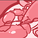Announcements
Applications are open for the 2024 Qlik Luminary Program. Apply by December 15 here.
cancel
Showing results for
Did you mean:Contributor III

## Value of a variable

Dear Community,

i have a switch-button to choose between day/week/month.

Now i want to get the corresponding field to use it in a bar chart for an aggregation.

How do i access this field?

The variables are defined in script as follows (it works fine)

LET v_sc_swt_select_intvk = 'day';
LET v_sc_swt_dim_intvk = 'if(''§(v_sc_swt_select_intvk)''=''month'',d_month_vk,if(''§(v_sc_swt_select_intvk)'' = ''week'',d_week_vk,if(''§(v_sc_swt_select_intvk)''=''day'',d_day_vk)))';
LET v_sc_swt_dim_intvk = Replace(v_sc_swt_dim_intvk,'§','\$');

within a barplot the height of bars shall be calculated with:

Sum(TOTAL <v_sc_swt_dim_intvk> qty_sold)*100

the variable v_sc_swt_dim_intvk should actually be e.g. d_day_vk.

What magic has to be done to display v_sc_swt_dim_intvk as the above defined fields

\$(v_sc_swt_dim_intvk) does not work